Custom SearchTANGENT AT A GIVEN POINT ON THE STANDARD PARABOLA The standard parabola is represented by the equation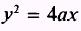Let P1 with coordinates (x1,y1) be a point on the curve. Choose P' on the curve, figure 3-4, near the given point so that the coordinates of P' are (x2,y2). As previously stated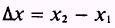Figure 3-4.-Parabola.   and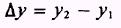so that by rearranging terms, the coordinates of P' may be written as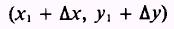Since P' is a point on the curve, y2 = 4ax the values of its coordinates may be substituted for x and y. This gives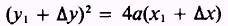or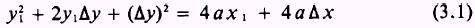The point P1(x1,y1) also lies on the curve, so we have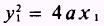Substituting this value for y; into equation (3.1) transforms it into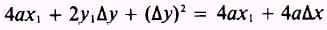Simplifying, we obtain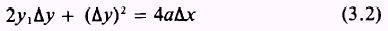Divide both sides by Ax, obtaining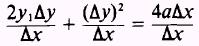which givesSolving for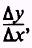we find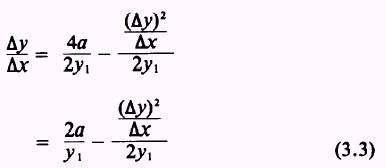Before proceeding, we need to discuss the term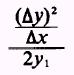in equation (3.3). If we solve equation (3.2) fory, we find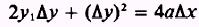then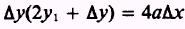andSince the denominator contains a term not dependent upony orx, as we letx approach zero,y will also approach zero. NOTE: We may find a value for Ax that will makey less than 1; then wheny is squared, it will approach zero at least as rapidly asx does. We now refer to equation (3.3) again and make the statement so that we may disregard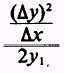, since it approaches zero when Ax approaches zero. ThenThe quantity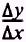is the slope of the line connecting P1 and P. From figure 3-4, the slope of the curve at P, is obviously different from the slope of the line connecting P1 and P'. Asx andy approach zero, the ratio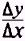will approach more and more closely the true slope of the curve at P1. We designate the slope by m. Thus, asx approaches zero, equation (3.4) becomes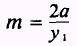The equation for a straight line in the point-slope form is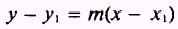Substituting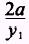for m gives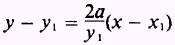Clearing fractions, we have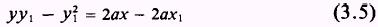but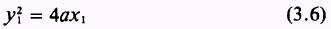Adding equations (3.5) and (3.6) yields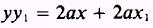Dividing by y1 giveswhich is an equation of a straight line in the slope-intercept form. This is the equation of the tangent line to the parabola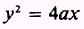at the point (x1,y1). EXAMPLE: Given the equationfind the slope of the curve and the equation of the tangent line at the point (2,4). SOLUTION:has the form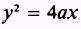where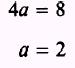and 2a=4 The slope, m, at point (2,4) becomesSince the slope of the line is 1, then the equation of the tangent to the curve at the point (2,4) is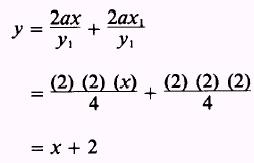Integrated Publishing, Inc. - A (SDVOSB) Service Disabled Veteran Owned Small Business